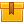# Using Multimeters

## Prerequisites

Knowledge of multimeter components and continuity checks is recommended.

## Description

This is the fourth lesson in the iKNOW® Multimeters Library. This lesson trains the learner to use a multimeter to measure resistance, AC voltage, DC voltage, current, frequency, and capacitance.

## Objectives

Use a multimeter to measure resistance, Measure AC voltage using a multimeter, Measure DC voltage using a multimeter, Use a multimeter to measure current, Use a multimeter to measure frequency and Use a multimeter to measure capacitance.

## ContentThis is the fourth lesson in the iKNOW™ Multimeters Library. This lesson
trains the learner to use a multimeter to measure resistance, AC
voltage, DC voltage, current, frequency, and capacitance.

•Using Multimeters

0
0 Reviews